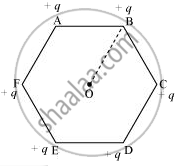# A regular hexagon of side 10 cm has a charge 5 µC at each of its vertices. Calculate the potential at the centre of the hexagon. - Physics

Numerical

A regular hexagon of side 10 cm has a charge 5 µC at each of its vertices. Calculate the potential at the centre of the hexagon.

#### Solution

The given figure shows six equal amounts of charges, q, at the vertices of a regular hexagon.Where,

Charge, q = 5 µC = 5 × 10−6 C

Side of the hexagon, l = AB = BC = CD = DE = EF = FA = 10 cm

Distance of each vertex from centre O, d = 10 cm

Electric potential at point O,

"V" = (6 xx "q")/(4piin_0"d")

Where,

in_0 = Permittivity of free space

1/(4piin_0) = 9 xx 10^9  "NC"^-2  "m"^-2

∴ "V" = (6 xx 9 xx 10^9 xx 5 xx 10^-6)/0.1

= 2.7 × 106 V

Therefore, the potential at the centre of the hexagon is 2.7 × 106 V.

Concept: Potential Energy of a System of Charges
Is there an error in this question or solution?

#### APPEARS IN

NCERT Physics Part 1 and 2 Class 12
Chapter 2 Electrostatic Potential and Capacitance
Exercise | Q 2.2 | Page 86
NCERT Class 12 Physics Textbook
Chapter 2 Electrostatic Potential and Capacitance
Exercise | Q 2 | Page 87
Share